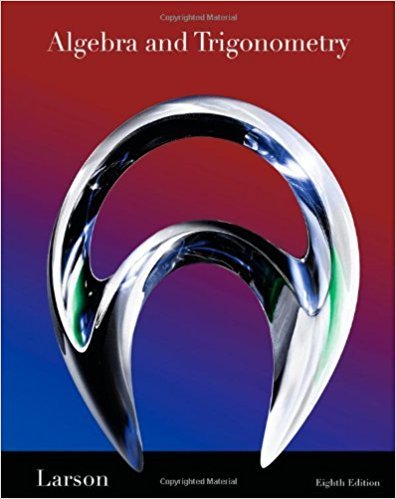×
×

# Solutions for Chapter 11.1: Sequences and Series## Full solutions for Algebra and Trigonometry | 8th Edition

ISBN: 9781439048474Solutions for Chapter 11.1: Sequences and Series

Solutions for Chapter 11.1
4 5 0 377 Reviews
25
5
##### ISBN: 9781439048474

Chapter 11.1: Sequences and Series includes 141 full step-by-step solutions. Since 141 problems in chapter 11.1: Sequences and Series have been answered, more than 51621 students have viewed full step-by-step solutions from this chapter. This expansive textbook survival guide covers the following chapters and their solutions. Algebra and Trigonometry was written by and is associated to the ISBN: 9781439048474. This textbook survival guide was created for the textbook: Algebra and Trigonometry, edition: 8.

Key Math Terms and definitions covered in this textbook
• Affine transformation

Tv = Av + Vo = linear transformation plus shift.

• Diagonal matrix D.

dij = 0 if i #- j. Block-diagonal: zero outside square blocks Du.

• Echelon matrix U.

The first nonzero entry (the pivot) in each row comes in a later column than the pivot in the previous row. All zero rows come last.

• Exponential eAt = I + At + (At)2 12! + ...

has derivative AeAt; eAt u(O) solves u' = Au.

• Graph G.

Set of n nodes connected pairwise by m edges. A complete graph has all n(n - 1)/2 edges between nodes. A tree has only n - 1 edges and no closed loops.

• Hilbert matrix hilb(n).

Entries HU = 1/(i + j -1) = Jd X i- 1 xj-1dx. Positive definite but extremely small Amin and large condition number: H is ill-conditioned.

• Inverse matrix A-I.

Square matrix with A-I A = I and AA-l = I. No inverse if det A = 0 and rank(A) < n and Ax = 0 for a nonzero vector x. The inverses of AB and AT are B-1 A-I and (A-I)T. Cofactor formula (A-l)ij = Cji! detA.

• Jordan form 1 = M- 1 AM.

If A has s independent eigenvectors, its "generalized" eigenvector matrix M gives 1 = diag(lt, ... , 1s). The block his Akh +Nk where Nk has 1 's on diagonall. Each block has one eigenvalue Ak and one eigenvector.

• Kirchhoff's Laws.

Current Law: net current (in minus out) is zero at each node. Voltage Law: Potential differences (voltage drops) add to zero around any closed loop.

• Kronecker product (tensor product) A ® B.

Blocks aij B, eigenvalues Ap(A)Aq(B).

• Least squares solution X.

The vector x that minimizes the error lie 112 solves AT Ax = ATb. Then e = b - Ax is orthogonal to all columns of A.

• Linear combination cv + d w or L C jV j.

• Lucas numbers

Ln = 2,J, 3, 4, ... satisfy Ln = L n- l +Ln- 2 = A1 +A~, with AI, A2 = (1 ± -/5)/2 from the Fibonacci matrix U~]' Compare Lo = 2 with Fo = O.

• Pivot columns of A.

Columns that contain pivots after row reduction. These are not combinations of earlier columns. The pivot columns are a basis for the column space.

• Row space C (AT) = all combinations of rows of A.

Column vectors by convention.

• Saddle point of I(x}, ... ,xn ).

A point where the first derivatives of I are zero and the second derivative matrix (a2 II aXi ax j = Hessian matrix) is indefinite.

• Sum V + W of subs paces.

Space of all (v in V) + (w in W). Direct sum: V n W = to}.

• Symmetric factorizations A = LDLT and A = QAQT.

Signs in A = signs in D.

• Trace of A

= sum of diagonal entries = sum of eigenvalues of A. Tr AB = Tr BA.

• Vector v in Rn.

Sequence of n real numbers v = (VI, ... , Vn) = point in Rn.

×